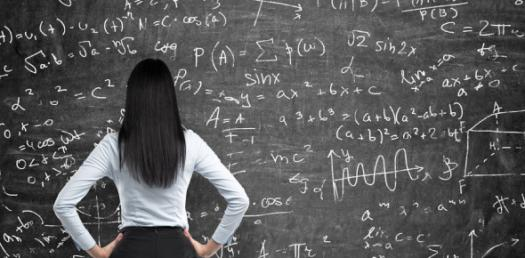12 Questions | Total Attempts: 5564262SettingsThe word Mathematics has evolved from the Greek word Mathema which means learning, knowledge, and study. There is no general definition of math and mathematicians use patterns to formulate new conjunctions until the truth has been resolved. The following quiz is meant for budding mathematicians. Good Luck!

• 1.
Who said "The universe can not be read until we learn the language and become familiar with the characters in which it is writte"?
• A.

Benjamin Peirc

• B.

Galileo Galilei

• C.

Festus Nelson

• D.

Jackson Peter

• 2.
Who referred to mathematics as the queen of science?
• A.

Carl Friedrich Gauss

• B.

Carl Peter John

• C.

John Peter

• D.

Benjamin Peirce

• 3.
........................ says as far as law of mathematics is reality, they are not certain and as far as they are certain they do not refer to reality?
• A.

Ahraham Bennett

• B.

Albert Einstein

• C.

Carl Gauss

• D.

Benjamin Peirce

• 4.
The history of mathematics can be seen as an ever increasing series of .............................?
• A.

Post

• B.

Figure

• C.

Set

• D.

Abstractions

• 5.
When did evidence of complex Mathematics start to appear?
• A.

3000 BC

• B.

2000 BC

• C.

4000 BC

• D.

5000 BC

• 6.
Where did the first elementary arithmetic first appear in records?
• A.

Archaeological

• B.

Mathematics

• C.

Algebra

• D.

Trigonometry

• 7.
Who invented Algebra?
• A.

Albraham Lincoln

• B.

Al Khwarizmi

• C.

Albert Einstein

• D.

Benjamin Peirce

• 8.
Where did the word Mathematic come from?
• A.

Ancient Golden Age

• B.

Ancient Greek

• C.

Ancient Egypt

• D.

Ancient Pakistan

• 9.
What is the name of the Italian mathematicians who introduced Hindu-Arabic numeral system?
• A.

Leonard Fibonacci

• B.

Aristotle

• C.

Abraham Licoln

• D.

Benjamin Peirce

• 10.
Mathematics arises from different type of .......................?
• A.

Algebra

• B.

Figures

• C.

Sets

• D.

Problems

• 11.
Pythagoras invented:
• A.

Pythagoras Laws of Mathematics

• B.

Pythagoras Theorem

• C.

Pythagoras Rules

• D.

Pythagoras Principles

• 12.
Aryabhata gave the world number "0". True or false?
• A.

True

• B.

FalseBack to top